# 《量化金融R语言高级教程》一1.2　波动率建模

+关注继续查看

### 1.2　波动率建模

getSymbols("SNP", from="2004-01-01", to=Sys.Date())
chartSeries(Cl(SNP))


ret <- dailyReturn(Cl(SNP), type='log')

par(mfrow=c(2,2))
acf(ret, main="Return ACF");
pacf(ret, main="Return PACF");
acf(ret^2, main="Squared return ACF");
pacf(ret^2, main="Squared return PACF")
par(mfrow=c(1,1))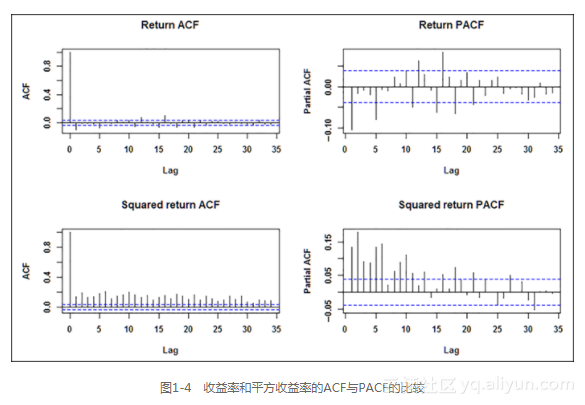m=mean(ret);s=sd(ret);
par(mfrow=c(1,2))
hist(ret, nclass=40, freq=FALSE, main='Return histogram');curve(dnorm(x,
mean=m,sd=s), from = -0.3, to = 0.2, add=TRUE, col="red")
plot(density(ret), main='Return empirical distribution');curve(dnorm(x,
mean=m,sd=s), from = -0.3, to = 0.2, add=TRUE, col="red")
par(mfrow=c(1,1))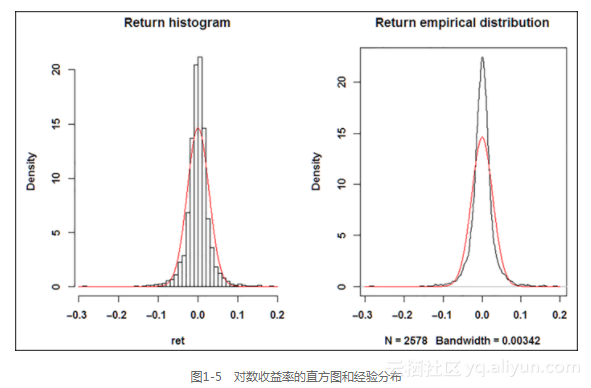> kurtosis(ret)
daily.returns
12.64　959


# 放大尾部
plot(density(ret), main='Return EDF - upper tail', xlim = c(0.1, 0.2),
ylim=c(0,2));
curve(dnorm(x, mean=m,sd=s), from = -0.3, to = 0.2, add=TRUE, col="red")


# 对数尺度上的密度图
plot(density(ret), xlim=c(-5*s,5*s),log='y', main='Density on log-scale')
curve(dnorm(x, mean=m,sd=s), from=-5*s, to=5*s, log="y", add=TRUE,
col="red")

# QQ-plot
qqnorm(ret);qqline(ret);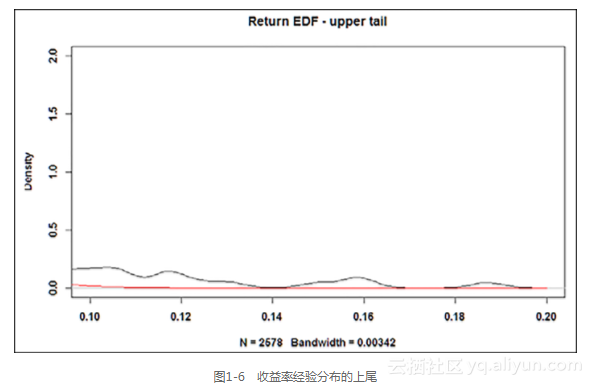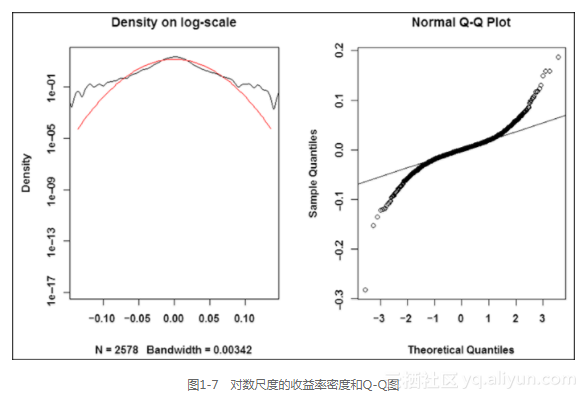### 1.2.1　通过rugarch包进行GARCH建模

R提供了多个包用于GARCH建模，其中最著名的包是rugarch包、rmgarch包（处理多变量模型）以及fGarch包。但是，基本的tseries包也包含了一些GARCH功能。在本章中，我们会展示rugarch包的建模能力。本章中的标记方法遵从rugarch包中各自的输出和文档惯例。

GARCH(p,q)过程可以如下表示：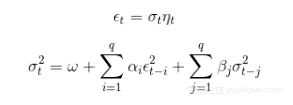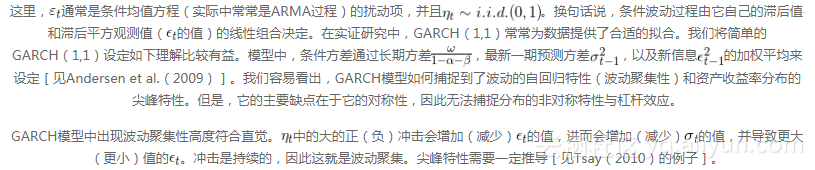install.packages('rugarch');library('rugarch')


#载入苹果股票数据并计算对数收益率
getSymbols("AAPL", from="2006-01-01", to="2014-03-31")
ret.aapl <- dailyReturn(Cl(AAPL), type='log')
chartSeries(ret.aapl)


rugrach包的编程逻辑可以如下理解：无论你的目标是什么（拟合、滤波、预测还是模拟），你首先需要指定一个模型作为系统对象（变量），再依次将它插入各个函数。模型可以通过调用ugrachspec()函数设定。下文的代码设定了一个简单的GARCH（1,1）模型（sGARCH），均值方程中仅有一个常数mu ：

garch11.spec = ugarchspec(variance.model = list(model="sGARCH",
garchOrder=c(1,1)), mean.model = list(armaOrder=c(0,0)))


aapl.garch11.fit = ugarchfit(spec=garch11.spec, data=ret.aapl)


> coef(aapl.garch11.fit)
mu　　　　omega　　　　　alpha1　　　　 beta1
1.923328e-03 1.027753e-05 8.191681e-02 8.987108e-01


coef(msft.garch11.fit) 　　  　　#估计的系数
vcov(msft.garch11.fit) 　　　  　#参数估计量的协方差矩阵
infocriteria(msft.garch11.fit) 　#常用信息量列表
newsimpact(msft.garch11.fit) 　　#计算信息冲击曲线
signbias(msft.garch11.fit) 　　　#Engle - Ng符号偏差检验
fitted(msft.garch11.fit) 　　  　#获得拟合的数据序列
residuals(msft.garch11.fit) 　 　#获得残差
uncvariance(msft.garch11.fit) 　　#无条件的（长期）方差
uncmean(msft.garch11.fit) 　　  　#无条件的（长期）均值


ni.garch11 <- newsimpact(aapl.garch11.fit)
plot(ni.garch11$zx, ni.garch11$zy, type="l", lwd=2, col="blue",
main="GARCH(1,1) - News Impact", ylab=ni.garch11$yexpr, xlab=ni. garch11$xexpr)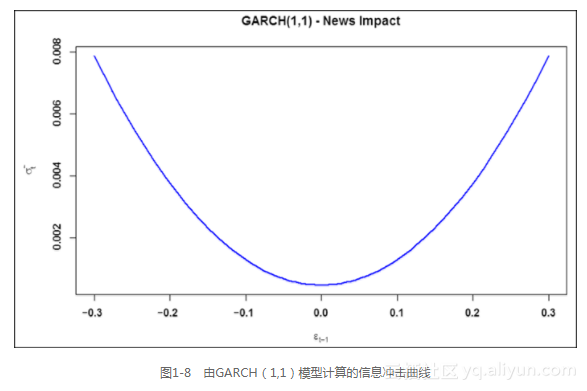Nelson（1991）提出了指数GARCH模型。这种方法直接对条件波动率的对数进行建模：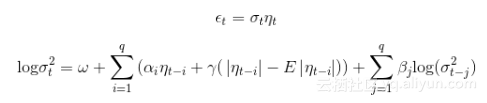# 指定在均值方程中只带有一个常数的EGARCH(1,1) 模型
egarch11.spec = ugarchspec(variance.model = list(model="eGARCH",
garchOrder=c(1,1)), mean.model = list(armaOrder=c(0,0)))
aapl.egarch11.fit = ugarchfit(spec=egarch11.spec, data=ret.aapl)

> coef(aapl.egarch11.fit)

mu　　　 omega　　　 alpha1　　　beta1　　　 gamma1
0.001446685 -0.291271433 -0.092855672 0.961968640 0.176796061


ni.egarch11 <- newsimpact(aapl.egarch11.fit)
plot(ni.egarch11$zx, ni.egarch11$zy, type="l", lwd=2, col="blue",
main="EGARCH(1,1) - News Impact",
ylab=ni.egarch11$yexpr, xlab=ni.egarch11$xexpr)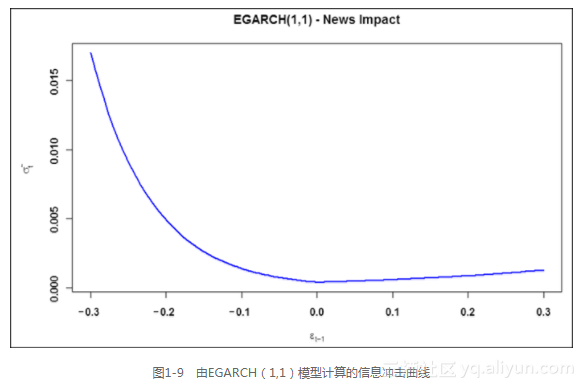TGARCH模型的方程式如下：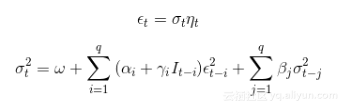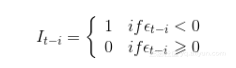# 指定在均值方程中只带有一个参数的TGARCH(1,1) 模型
tgarch11.spec = ugarchspec(variance.model = list(model="fGARCH",
submodel="TGARCH", garchOrder=c(1,1)),
mean.model = list(armaOrder=c(0,0)))
aapl.tgarch11.fit = ugarchfit(spec=tgarch11.spec, data=ret.aapl)

> coef(aapl.egarch11.fit)f
mu　　　　 omega　　　　 alpha1　　beta1　　　 gamma1
0.001446685 -0.291271433 -0.092855672 0.961968640 0.176796061


ni.tgarch11 <- newsimpact(aapl.tgarch11.fit)
plot(ni.tgarch11$zx, ni.tgarch11$zy, type="l", lwd=2, col="blue",
main="TGARCH(1,1) - News Impact",
ylab=ni.tgarch11$yexpr, xlab=ni.tgarch11$xexpr)
<span style='display:block;text-align:center'>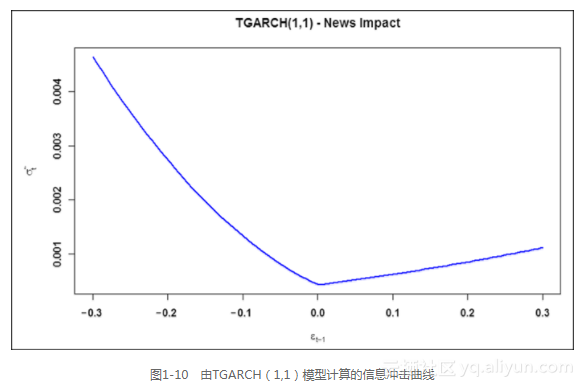### 1.2.2　模拟和预测

rugarch包允许从指定的模型以一种简单的方式模拟。当然，为了模拟，我们还需指定ugarchspec()内的模型参数，这可以通过fixed.pars参数完成。指定GARCH模型和给定条件均值之后，仅用ugarchpath()函数，就可以模拟出一个相应的时间序列：

garch11.spec = ugarchspec(variance.model = list(garchOrder=c(1,1)),
mean.model = list(armaOrder=c(0,0)),
fixed.pars=list(mu = 0, omega=0.1, alpha1=0.1,
beta1 = 0.7))
garch11.sim = ugarchpath(garch11.spec, n.sim=1000)


aapl.garch11.fit = ugarchfit(spec=garch11.spec, data=ret.aapl, out.
sample=20)
n.roll=10)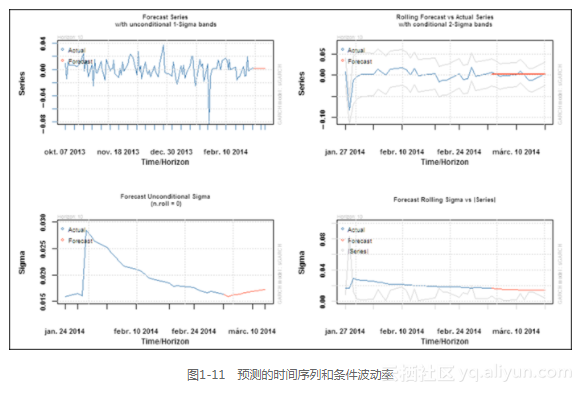现在的技术真的很厉害，iPhone都能山寨几乎一样，外观不用说，系统UI都做得差不多相同，ytkah的一位朋友之前就被人骗了，她拿来手机让我优化，说是很卡，起初ytkah也琢磨很久，只是持怀疑态度，没发现什么破绽，后面在iTunes那边安装软件时才发现了端倪。
698 0《量化金融R语言高级教程》一1.3　小结
1317 0《量化金融R语言高级教程》一1.2　波动率建模
5927 0耗电量大是大多数智能手机的痛，好玩的东西多了，电量也撑不了多久。手机快没电时如何快速发送微信位置给好友好让他容易找到你呢？微信就内藏这样的彩蛋。 　　在微信任意聊天窗口输入 //pickpoi 并发送，可以快速定位自己当前的位置！当然这也与微信中的“发送位置”的功能是基本一致的。
746 0如果你用的是苹果版微信，那么恭喜你，当微信使用久后出现故障或者异常，比如聊天记录混乱、通讯录丢失等，可以一键修复了。怎么操作呢？输入一个指令就可以实现。 　　如下图点击微信首页右上角的号，选择“添加朋友”，然后在添加朋友界面中输入   :recover （注意有冒号哟）。
689 0Oracle 10g 高级安装图文教程(二）
781 0《量化金融R语言高级教程》一1.4　参考文献
1644 0有时我们出门在外难免会出现网络信号不好的时候，微信会提示“无法连接到服务器”，可能还会弹出一个“诊断网络”的按钮窗口。要是没弹出怎么弄呢？其实微信早就藏着这个彩蛋了，我们没挖掘到而已。 　　在微信任意聊天窗口输入 //traceroute 并发送，还可以调出“诊断网络”功能。
1582 0《Java大学教程》—第18章 高级图形编程
1113 0虽然愚人节已经过去好长一段时间了，但好友之间的整蛊游戏不会就这样结束。看好了，微信整人游戏高级版来了：在微信任意聊天窗口输入 //fullexit ，相当于退出功能，输入并发送后，微信会自动闪退到桌面！再次进入微信时，还需要输入登录密码！假使他真忘记密码不要来找ytkah哈，我也不知道他的微信密码。
963 0

12049

0

JS零基础入门教程（上册）